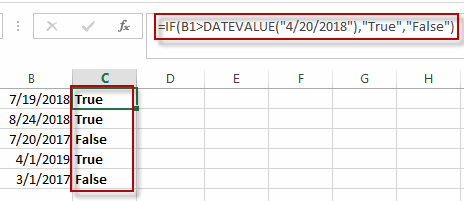# Compare Dates

This post will guide you how to compare dates if it is greater than a given date in excel. How do I compare a list of dates with a specified date using a formula in Excel.

## Compare Dates

Assuming that you have a list of data that contain date values in Excel, you can use the IF function to create a formula to achieve it. If the date is greater that the given date value, then return True. Otherwise, it returns False.

The formula is like as below:

=IF(B1>DATEVALUE(“4/20/2018″),”True”,”False”)

Type this formula into a blank cell and then press Enter key in your keyboard, and then drag the AutoFill Handle over other cells to apply this formula to compare dates.The DATEVALUE function will convert the date to a serial number. So that if can do the comparing operation. And the IF function will compare the date value in the cell B1 with the serial number returned by the DATEVALUE function.

### Related Functions

• Excel IF function
The Excel IF function perform a logical test to return one value if the condition is TRUE and return another value if the condition is FALSE. The IF function is a build-in function in Microsoft Excel and it is categorized as a Logical Function.The syntax of the IF function is as below:= IF (condition, [true_value], [false_value])….
• Excel DATEVALUE Function
The Excel DATEVALUE function returns the serial number of date. And it can be used to convert a date represented as text format into a serial number that recognizes as a date format.The syntax of the DATEVALUE function is as below:=DATEVALUE(date_text)…